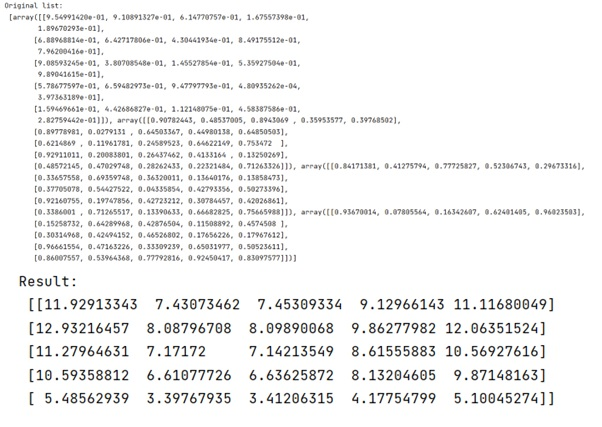# Multiply several matrices in numpy

Learn, how to multiply several matrices in numpy at once?
Submitted by Pranit Sharma, on February 16, 2023

NumPy is an abbreviated form of Numerical Python. It is used for different types of scientific operations in python. Numpy is a vast library in python which is used for almost every kind of scientific or mathematical operation. It is itself an array which is a collection of various methods and functions for processing the arrays.

## NumPy - Multiplying several matrices

Suppose that we are given N square matrices (M1...Mn). we need to find a way to multiply these matrices in a neat way.

For this purpose, we will use the reduce function along with the dot product. Reduce function multiplies two matrices at a time and then reduces the result to multiply it with the other variables passed inside it.

Let us understand with the help of an example,

## Python code to multiply several matrices in numpy

```# Import numpy
import numpy as np

# Import functools
import functools

# Creating a list of numpy matrices
A = [np.random.random((5, 5)) for i in range(4)]

# Display original list
print("Original list:\n",A,"\n")

# Calculating dot product of all the matrices
res = functools.reduce(np.dot, A)

# Display result
print("Result:\n",res)
```

Output: